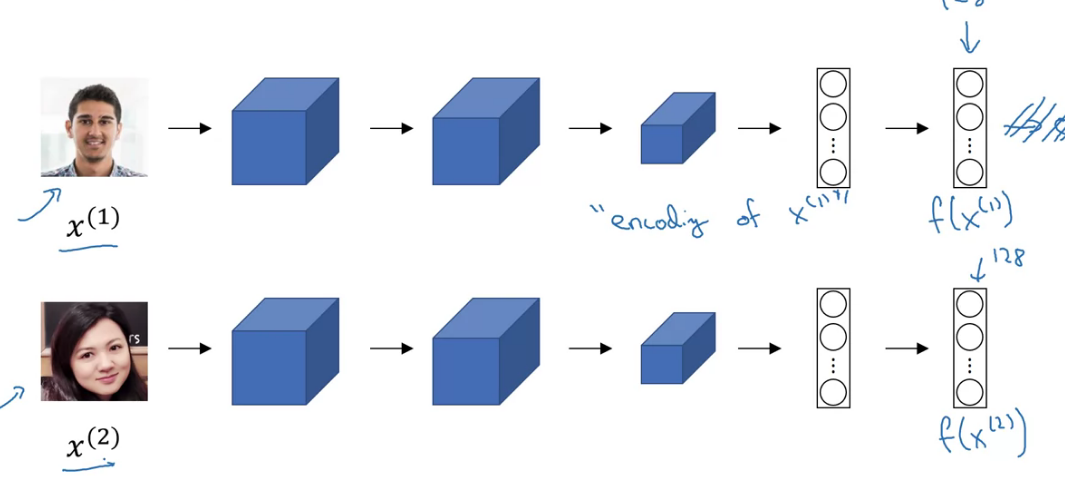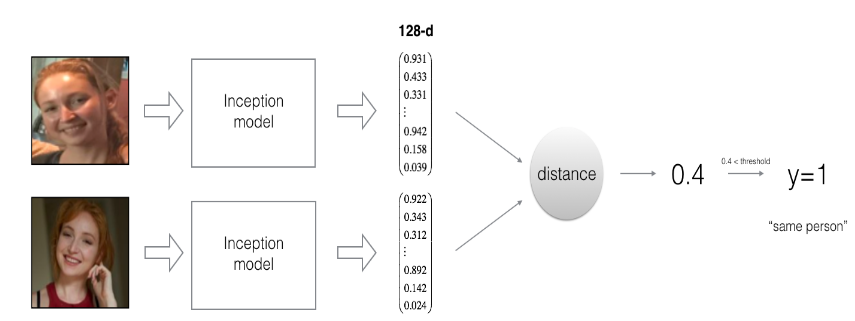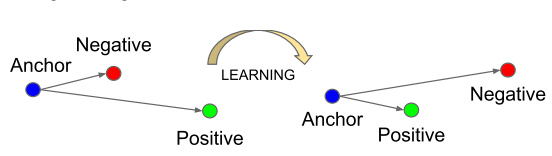# Face recognition - Class Review

Last updated on：a year ago

Face verification requires comparing a new picture against one person’s face, whereas face recognition requires comparing a new picture against K person’s faces. However, both of them are based on the same theory.

# What is face recognition

Face recognition is widely used in verification and recognition.

Verification

• Input image name/ID
• Output whether the input image is that of the claimed person

Recognition

• Has a database of K person
• Get an input image
• Output ID if the image is any of the K persons (or “not recognized”)

Differences

• Face verification solves an easier 1:1 matching problem; face recognition addresses a harder 1:K matching problem.
• The same encoding can be used for verification and recognition. Measuring distances between two images’ encodings allows you to determine whether they are pictures of the same person.

# One-shot learning

Learn from one example to recognize the person again. eg. 5 person then 6 output (softmax)

Learning a “similarity” function

For verification,

$$d(img1, img2) = \text{degree of difference between images}$$

$$\text{If} d(img1, img2) \begin{bmatrix} \le \tau \text{“same”} \\ \ge \tau \text{“same”} \\ \end{bmatrix}$$

## Q&A

2.Why do we learn a function d(img1, img2)d(img1,img2) for face verification? (Select all that apply.) Given how few images we have per person, we need to apply transfer learning.

-[x] We need to solve a one-shot learning problem.

-[x] This allows us to learn to recognize a new person given just a single image of that person.

-[ ] This allows us to learn to predict a person’s identity using a softmax output unit, where the number of classes equals the number of persons in the database plus 1 (for the final “not in database” class).

The methodology of final choice is used in face recognition.

# Siamese network

The upper and lower neural networks have different input images, but have exactly the same parameters.$$d(x^{(1)}, (x^{(2)}) = \Vert{f(x^{(1)}) -f(x^{(2)})}\Vert ^2_2$$

Parameters of NN define an encoding $f(x^{(i)})$
Learn parameters so that:
If $x^{(i)}, x^{(j)}$ are the same person, $\Vert {f(x^{(1)}) -f(x^{(2)})} \Vert^2$ is small,
If $x^{(i)}, x^{(j)}$ are the same person, $\Vert {f(x^{(1)}) -f(x^{(2)})} \Vert^2$ is large.

# Triplet Loss

Triplet loss is an effective loss function for training a neural network to learn an encoding of a face image.
Training will use triplets of images $(A, P, N)$:

• A is an “Anchor” image–a picture of a person.
• P is a “Positive” image–a picture of the same person as the Anchor image.
• N is a “Negative” image–a picture of a different person than the Anchor image.
You’d like to make sure that an image $A^{(i)}$ of an individual is closer to the Positive $P^{(i)}$ than to the Negative image $N^{(i)}$) by at least a margin $\alpha$:

$$\Vert f\left(A^{(i)}\right) -f \left(P^{(i)}\right) \Vert_{2}^{2} \alpha< \Vert f \left(A^{(i)} \right) - f \left(N^{(i)}\right) \Vert_{2}^{2}$$

$$\Vert f(A) -f(P) \Vert^2 -\Vert f(A) - f(N)\Vert^2 + \alpha <= 0$$

$\alpha$ is here to enforce a margin between the positive and the negatives.

Given 3 images A, P, N, loss function:
$$L(A, P, N) = max(\Vert f(A) -f(P)\Vert^2 -\Vert f(A) - f(N)\Vert^2 + \alpha, 0)$$
$$J = \sum^m_{i=1} L(A^{(i)}, P^{(i)}, N^{(i)})$$
Or,
$$\mathcal{J} = \sum^{m}_{i=1} \large[ \small \underbrace{\mid \mid f(A^{(i)}) - f(P^{(i)}) \mid \mid_2^2}_\text{(1)} - \underbrace{\mid \mid f(A^{(i)}) - f(N^{(i)}) \mid \mid_2^2}_\text{(2)} + \alpha \large ] \small_+ \tag{3}$$
Here, the notation “$[z]_+$” is used to denote $max(z,0)$.

Note

• The term (1) is the squared distance between the anchor “A” and the positive “P” for a given triplet; you want this to be small.
• The term (2) is the squared distance between the anchor “A” and the negative “N” for a given triplet, you want this to be relatively large. It has a minus sign preceding it because minimizing the negative of the term is the same as maximizing that term.
• $\alpha$ is called the margin. It’s a hyperparameter that you pick manually. You’ll use $\alpha = 0.2$.

Choosing the triplets A, P, N
During training, if A, P, N are chosen randomly, $d(A, P) + \alpha <= d(A, N)$ is easily satisfied
solution: choose triplets that’re “hard” to train on
$$d(A, P) + \alpha \le d(A, N)$$
$$d(A, P) \approx d(A, N)$$

Here we want to ensure that an image $x^a_i$ (anchor) of a specific person is closer to all other images $x^p_i$ (positive) of the same person than it is to any image $x^n_i$ (negative) of any other person.Face verification and binary classification

$$\hat{y} = \sigma (\sum^{128}_{k=1} w_k \vert f(x^{(i)})_k - f(x^{(j)})_k \vert + b) \ \text{or} \ (f(x^{(i)}) _k - f(x^{(j)}) _k)^2$$

# Ways to improve

• Put more images of each person (under different lighting conditions, taken on different days, etc.) into the database. Then, given a new image, compare the new face to multiple pictures of the person. This would increase accuracy.
• Crop the images to contain just the face, and less of the “border” region around the face. This pre-processing removes some of the irrelevant pixels around the face, and also makes the algorithm more robust.

# Reference

 Schroff, F., Kalenichenko, D. and Philbin, J., 2015. Facenet: A unified embedding for face recognition and clustering. In Proceedings of the IEEE conference on computer vision and pattern recognition (pp. 815-823).

 Deeplearning.ai, Convolutional Neural Networks